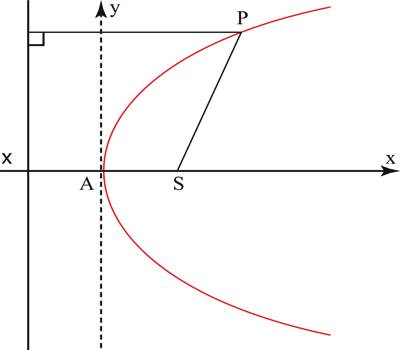Parabola in Coordinate Geometry

Co-ordinates Geometry is a system of geometry where the position of points on the plane is described using an ordered pair of numbers. Straight lines in coordinate geometry are the same idea as in regular geometry, except that they are drawn on a coordinate plane and we can do more with them.

A parabola is one of the Conics. These are defined by their focus-directrix property, i.e. as the locus of a point which moves so that its distance from a fixed point is in a constant ratio to its distance from a fixed line.

The fixed point is called the “Focus”, the fixed line the “Directerix” and the constant ratio the “Eccentricity”.(i) Standard equation of parabola is y2 = 4ax. Its vertex is the origin and axis is x-axis.

(ii) Other forms of the equations of parabola:

(a) x2 = 4ay. Its vertex is the origin and axis is y-axis.

(b) (y – β)2 = 4a (x – α). Its vertex is at (α, β) and axis is parallel to x-axis.

(c) (x – α)2 = 4a(y- β). Its vertex is at ( a, β) and axis is parallel to y-axis.

(iii) x = ay2 + by + c (a ≠ o) represents equation of the parabola whose axis is parallel to x-axis.

(iv) y = px2 + qx + r (p ≠ o) represents equation of the parabola whose axis is parallel to y-axis.

(v) The parametric equations of the parabola y2 = 4ax are x = at2 , y = 2at, t being the parameter.

(vi) The point (x1, y1) lies outside, on or inside the parabola y2 = 4ax according as y12 = 4ax1 >, = or,<0

Information Source: